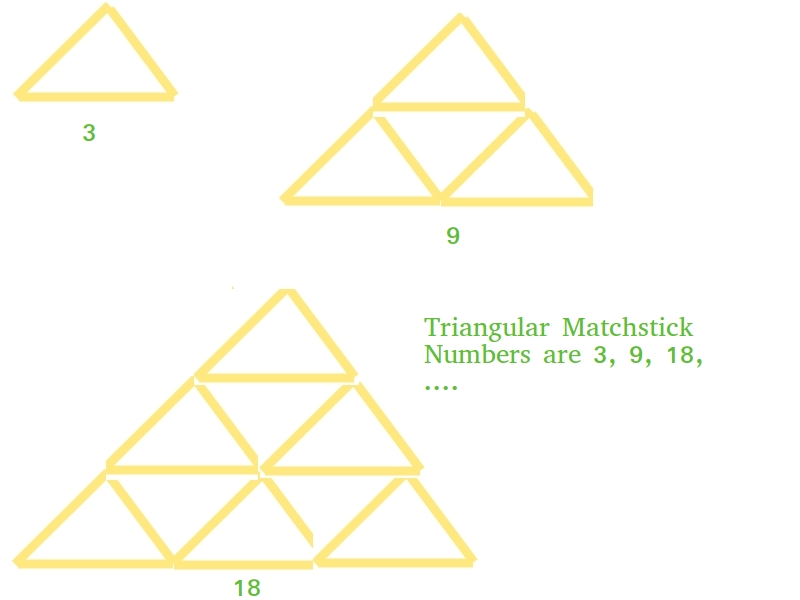# C/C++ Program for Triangular Matchstick Number

Given a number X which represents the floor of a matchstick pyramid, write a program to print the total number of matchstick required to form a pyramid of matchsticks of x floors.

Examples:

```Input : X = 1
Output : 3
Input : X = 2
Output : 9
```

## Recommended: Please try your approach on {IDE} first, before moving on to the solution.

This is mainly an extension of triangular numbers. For a number X, the matchstick required will be three times of X-th triangular numbers, i.e., (3*X*(X+1))/2`// C++ program to find X-th triangular ` `// matchstick number ` ` `  `#include ` `using` `namespace` `std; ` ` `  `int` `numberOfSticks(``int` `x) ` `{ ` `    ``return` `(3 * x * (x + 1)) / 2; ` `} ` ` `  `int` `main() ` `{ ` `    ``cout << numberOfSticks(7); ` `    ``return` `0; ` `} `

Output:

```84
```

Please refer complete article on Triangular Matchstick Number for more details!

My Personal Notes arrow_drop_up
Article Tags :

Be the First to upvote.

Please write to us at contribute@geeksforgeeks.org to report any issue with the above content.